Electron. J. Diff. Eqns., Vol. 2001(2001), No. 54, pp. 1-18.

### Asymptotic behaviour for Schrodinger equations with a quadratic nonlinearity in one-space dimension Nakao Hayashi & Pavel I. Naumkin

Abstract:
We consider the Cauchy problem for the Schr\"{o}dinger equation with a quadratic nonlinearity in one space dimension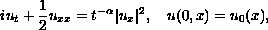where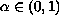. From the heuristic point of view, solutions to this problem should have a quasilinear character when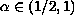. We show in this paper that the solutions do not have a quasilinear character for all. due to the special structure of the nonlinear term. We also prove that for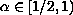if the initial data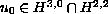are small, then the solution has a slow time decay such as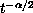. For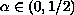, if we assume that the initial data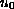are analytic and small, then the same time decay occurs.

Submitted May 22, 2001. Published July 25, 2001.
Math Subject Classifications: 35Q55, 74G10, 74G25.
Key Words: Schrodinger equation, large time behaviour, quadratic nonlinearity.

Show me the PDF file (302K), TEX file, and other files for this article.Nakao Hayashi Department of Mathematics Graduate School of Science Osaka University Toyonaka, Osaka 560-0043, Japan e-mail : nhayashi@math.wani.osaka-u.ac.jp Pavel I. Naumkin Instituto de Fisica y Matematicas Universidad Michoacana, AP 2-82 Morelia, CP 58040, Michoacan, Mexico e-mail: pavelni@zeus.ccu.umich.mx